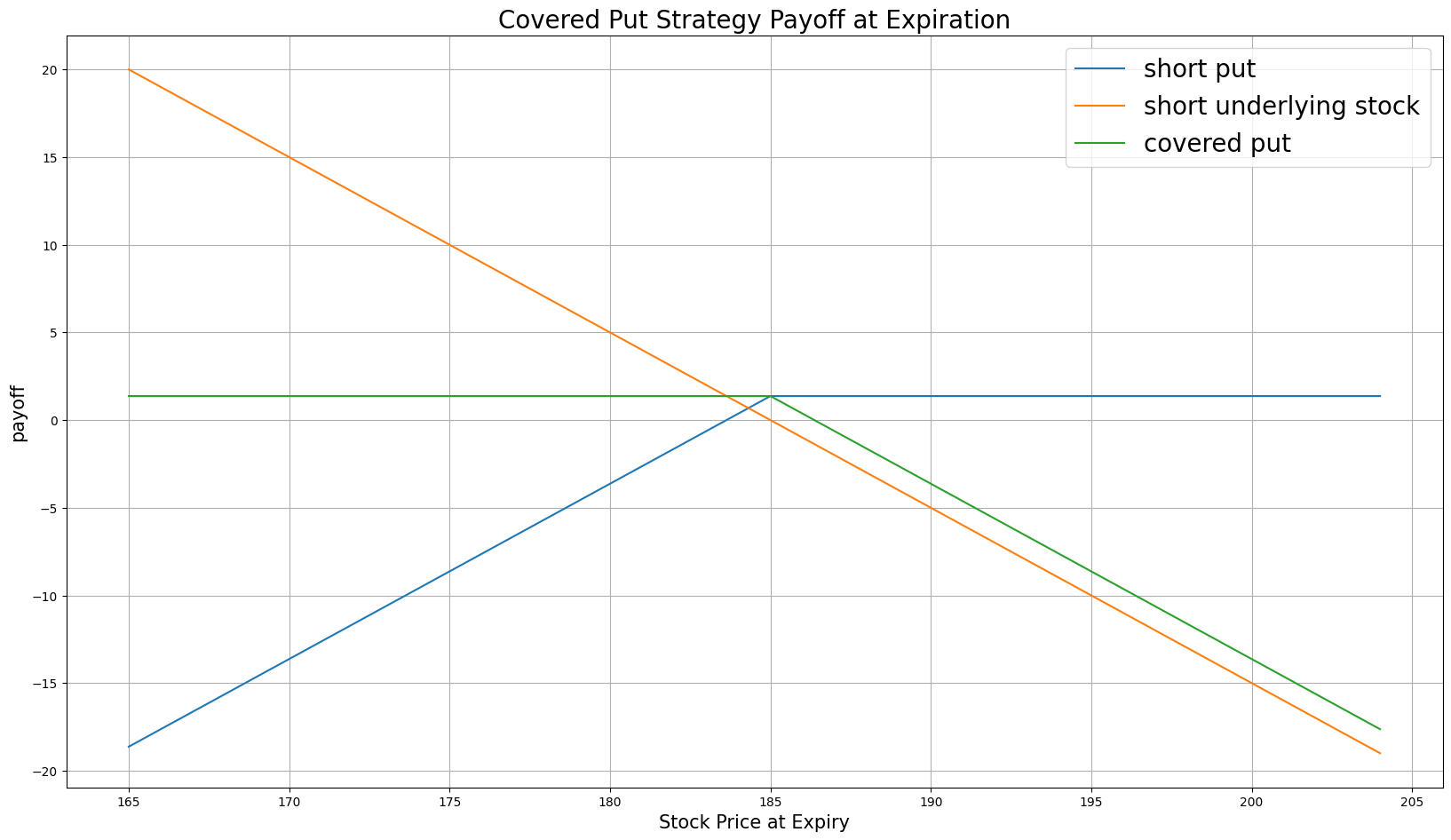# Option Strategies

## Covered Put

### Introduction

A Covered Put consists of a short position in a stock and a short position in put Options for the same amount of stock. Covered puts aim to profit from the Option premium by selling puts written on the stock you already shorted. At any time for American Options or at expiration for European Options, if the stock moves below the strike price, you keep the premium and still maintain the underlying Equity position. If the underlying price moves above the strike, the Option buyer can exercise the Options contract, which mean you buy the stock at the strike price but you will still keep the premium. Another risk of a covered put comes from the short stock position, which can drop in value.

### Implementation

Follow these steps to implement the covered put strategy:

1. In the Initialize method, set the start date, end date, starting cash, and Options universe.
2. private Symbol _put, _symbol;

public override void Initialize()
{
SetStartDate(2014, 1, 1);
SetEndDate(2014, 3, 1);
SetCash(100000);

_symbol = option.Symbol;
option.SetFilter(-3, 3, 0, 31);
}
def Initialize(self) -> None:
self.SetStartDate(2014, 1, 1)
self.SetEndDate(2014, 3, 1)
self.SetCash(100000)

self.symbol = option.Symbol
self.put = None

option.SetFilter(-3, 3, 0, 31)
3. In the OnData method, select the Option contract.
4. public override void OnData(Slice slice)
{
if (_put != null && Portfolio[_put].Invested) return;

if (!slice.OptionChains.TryGetValue(_symbol, out var chain)) return;

// Find ATM put with the farthest expiry
var expiry = chain.Max(x => x.Expiry);
var atmput = chain
.Where(x => x.Right == OptionRight.Put && x.Expiry == expiry)
.OrderBy(x => Math.Abs(x.Strike - chain.Underlying.Price))
.FirstOrDefault();
def OnData(self, slice: Slice) -> None:
if self.put and self.Portfolio[self.put].Invested:
return

chain = slice.OptionChains.get(self.symbol)
if not chain:
return

# Find ATM put with the farthest expiry
expiry = max([x.Expiry for x in chain])
put_contracts = sorted([x for x in chain
if x.Right == OptionRight.Put and x.Expiry == expiry],
key=lambda x: abs(chain.Underlying.Price - x.Strike))

if not put_contracts:
return

atm_put = put_contracts
5. In the OnData method, put the OptionStrategies.CoveredPut method and then submit the order.
6. var coveredput = OptionStrategies.CoveredPut(_symbol, atmput.Strike, expiry);

_put = atmput.Symbol;
covered_put = OptionStrategies.CoveredPut(self.symbol, atm_put.Strike, expiry)

self.put = atm_put.Symbol

Option strategies synchronously execute by default. To asynchronously execute Option strategies, set the asynchronous argument to Falsefalse. You can also provide a tag and order properties to the Buy method.

Buy(optionStrategy, quantity, asynchronous, tag, orderProperties);

self.Buy(option_strategy, quantity, asynchronous, tag, order_properties)


### Strategy Payoff

The payoff of the strategy is

$$\begin{array}{rcll} P^{K}_T & = & (K - S_T)^{+}\\ P_T & = & (S_0 - S_T + P^{K}_0 - P^{K}_T)\times m - fee \end{array}$$ $$\begin{array}{rcll} \textrm{where} & P^{K}_T & = & \textrm{Put value at time T}\\ & S_T & = & \textrm{Underlying asset price at time T}\\ & K & = & \textrm{Put strike price}\\ & P_T & = & \textrm{Payout total at time T}\\ & S_0 & = & \textrm{Underlying asset price when the trade opened}\\ & P^{K}_0 & = & \textrm{Put price when the trade opened (credit received)}\\ & m & = & \textrm{Contract multiplier}\\ & T & = & \textrm{Time of expiration} \end{array}$$

The following chart shows the payoff at expiration:The maximum profit is $S_T - K + P^{K}_0$. It occurs when the underlying price is at or below the strike price of the put at expiration.

If the underlying price increase, the maximum loss is unlimited.

If the Option is American Option, there is risk of early assignment on the sold contract.

### Example

The following table shows the price details of the assets in the algorithm:

AssetPrice ($)Strike ($)
Put1.37185.00
Underlying Equity at start of the trade186.94-
Underlying Equity at expiration190.01-

Therefore, the payoff is

$$\begin{array}{rcll} P^{K}_T & = & (K - S_T)^{+}\\ & = & (185 - 190.01)^{+}\\ & = & 0\\ P_T & = & (S_0 - S_T + P^{K}_0 - P^{K}_T)\times m - fee\\ & = & (186.94 - 190.01 + 1.37 - 0)\times m - fee\\ & = & -1.70 \times 100 - 2\\ & = & -172 \end{array}$$

So, the strategy loses \$172.

The following algorithm implements a covered put strategy:

You can also see our Videos. You can also get in touch with us via Discord.